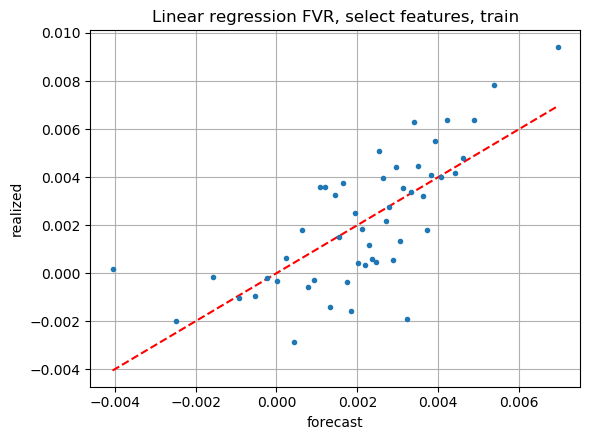# Ordinary least squares linear regression

Multiple regression is the ubiquitous workhorse of most scientific fields. The solution form is interpretable. It is very efficient to solve due to the simple closed-form solution. For massive datasets, finding the solution can even be parallelized using map-reduce.

## Weighted multivariate regression

In matrix-vector form, we are solving:

$$\min_{\boldsymbol{\alpha}} \bold{w}' (\bold{y} - \bold{X} \boldsymbol{\alpha})^2$$

where:

• $\bold{X}$ is the $m \times n$ design matrix, with $m$ samples and $n$ features. I did not include a column of ones, as it would be a linear combination of other features
• $\bold{y}$ is the $m \times 1$ vector of targets, or the dependent variable
• $\bold{w}$ is the $m \times 1$ vector of sample weights
• $\boldsymbol{\alpha}$ is the $n \times 1$ vector of solution coefficients

Take the derivative, set it equal to zero, and the solution is:

$$\boldsymbol{\alpha} = (\bold{X}' \bold{W} \bold{X})^{-1} \bold{X}'\bold{W} \bold{y}$$

Where $\bold{W}$ is a diagonal matrix of the vector $\bold{w}$. Inference is done like this:

$$\bold{\hat{y}} = \bold{X} \boldsymbol{\alpha}$$

## Sample code

The relevant part of my python code for this post is very short. I used scikit-learn:

from sklearn.linear_model import LinearRegression

model = LinearRegression(fit_intercept=False)
model.fit(train_X, train_y, sample_weight=train_w)

train_pred = model.predict(train_X)
test_pred = model.predict(test_X)


## Dataset-1 model, all features

On dataset-1, the linear model with all features looks great on the training data FVR plot. I like that it has 8 (out of 50) dots to the right of 0.005 on the x-axis, whereas my handcrafted model only has 4.

The out-of-sample performance isn’t very good, though. I prefer the out-of-sample performance of my hand-crafted model.

## Dataset-1 model, select features

Out of curiosity, what if I only let it train on the 5 features I ended up using on my handcrafted model? Here are the FVR plots:As expected, the model looks worse on the training data. On the test data, it’s still not great, but perhaps better than the model trained on all features.